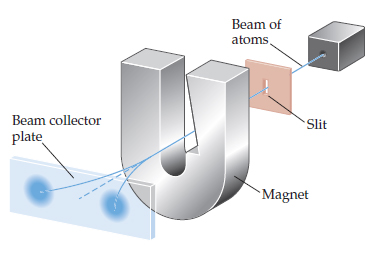# Problem: In the experiment shown schematically below , a beam of neutral atoms is passed through a magnetic field. Atoms that have unpaired electrons are deflected in different directions in the magnetic field depending on the value of the electron spin quantum number. In the experiment illustrated, we envision that a beam of hydrogen atoms splits into two beams.What do you think would happen if the strength of the magnet were increased?

###### FREE Expert Solution

What we are talking about here is paramagnetism.

Anything that is magnetic, like a bar magnet or a loop of electric current, has a magnetic moment.

85% (44 ratings)###### Problem Details

In the experiment shown schematically belowa beam of neutral atoms is passed through a magnetic field. Atoms that have unpaired electrons are deflected in different directions in the magnetic field depending on the value of the electron spin quantum number. In the experiment illustrated, we envision that a beam of hydrogen atoms splits into two beams.

What do you think would happen if the strength of the magnet were increased?

Frequently Asked Questions

What scientific concept do you need to know in order to solve this problem?

Our tutors have indicated that to solve this problem you will need to apply the Quantum Numbers: Spin Quantum Number concept. You can view video lessons to learn Quantum Numbers: Spin Quantum Number. Or if you need more Quantum Numbers: Spin Quantum Number practice, you can also practice Quantum Numbers: Spin Quantum Number practice problems.

What professor is this problem relevant for?

Based on our data, we think this problem is relevant for Professor Smith's class at MSSTATE.